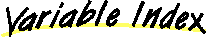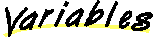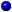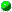Class java.lang.Double
`All Packages  Class Hierarchy  This Package  Previous  Next  Index`

# Class java.lang.Double

```java.lang.Object
|
+----java.lang.Number
|
+----java.lang.Double
```

public final class Double
extends Number
The Double class provides an object wrapper for Double data values and serves as a place for double-oriented operations. A wrapper is useful because most of Java's utility classes require the use of objects. Since doubles are not objects in Java, they need to be "wrapped" in a Double instance.

##MAX_VALUE
The maximum value a double can have.MIN_VALUE
The minimum value a double can have.NEGATIVE_INFINITY
Negative infinity.NaN
Not-a-Number.POSITIVE_INFINITY
Positive infinity.

##Double(double)
Constructs a Double wrapper for the specified double value.Double(String)
Constructs a Double object initialized to the value specified by the String parameter.

##doubleToLongBits(double)
Returns the bit represention of a double-float valuedoubleValue()
Returns the double value of this Double.equals(Object)
Compares this object against the specified object.floatValue()
Returns the float value of this Double.hashCode()
Returns a hashcode for this Double.intValue()
Returns the integer value of this Double (by casting to an int).isInfinite(double)
Returns true if the specified number is infinitely large in magnitude.isInfinite()
Returns true if this Double value is infinitely large in magnitude.isNaN(double)
Returns true if the specified number is the special Not-a-Number (NaN) value.isNaN()
Returns true if this Double value is the special Not-a-Number (NaN) value.longBitsToDouble(long)
Returns the double-float corresponding to a given bit represention.longValue()
Returns the long value of this Double (by casting to a long).toString(double)
Returns a String representation for the specified double value.toString()
Returns a String representation of this Double object.valueOf(String)
Returns a new Double value initialized to the value represented by the specified String.

##POSITIVE_INFINITY
```  public final static double POSITIVE_INFINITY
```
Positive infinity.NEGATIVE_INFINITY
```  public final static double NEGATIVE_INFINITY
```
Negative infinity.NaN
```  public final static double NaN
```
Not-a-Number. Note: is not equal to anything, including itselfMAX_VALUE
```  public final static double MAX_VALUE
```
The maximum value a double can have. The greatest maximum value that a double can have is 1.79769313486231570e+308d.MIN_VALUE
```  public final static double MIN_VALUE
```
The minimum value a double can have. The lowest minimum value that a double can have is 4.94065645841246544e-324d.

##Double
```  public Double(double value)
```
Constructs a Double wrapper for the specified double value.
Parameters:
value - the initial value of the doubleDouble
```  public Double(String s) throws NumberFormatException
```
Constructs a Double object initialized to the value specified by the String parameter.
Parameters:
s - the String to be converted to a Double
Throws: NumberFormatException
If the String does not contain a parsable number.

##toString
```  public static String toString(double d)
```
Returns a String representation for the specified double value.
Parameters:
d - the double to be convertedvalueOf
```  public static Double valueOf(String s) throws NumberFormatException
```
Returns a new Double value initialized to the value represented by the specified String.
Parameters:
s - the String to be parsed
Throws: NumberFormatException
If the String cannot be parsed.isNaN
```  public static boolean isNaN(double v)
```
Returns true if the specified number is the special Not-a-Number (NaN) value.
Parameters:
v - the value to be testedisInfinite
```  public static boolean isInfinite(double v)
```
Returns true if the specified number is infinitely large in magnitude.
Parameters:
v - the value to be testedisNaN
```  public boolean isNaN()
```
Returns true if this Double value is the special Not-a-Number (NaN) value.isInfinite
```  public boolean isInfinite()
```
Returns true if this Double value is infinitely large in magnitude.toString
```  public String toString()
```
Returns a String representation of this Double object.
Overrides:
toString in class ObjectintValue
```  public int intValue()
```
Returns the integer value of this Double (by casting to an int).
Overrides:
intValue in class NumberlongValue
```  public long longValue()
```
Returns the long value of this Double (by casting to a long).
Overrides:
longValue in class NumberfloatValue
```  public float floatValue()
```
Returns the float value of this Double.
Overrides:
floatValue in class NumberdoubleValue
```  public double doubleValue()
```
Returns the double value of this Double.
Overrides:
doubleValue in class NumberhashCode
```  public int hashCode()
```
Returns a hashcode for this Double.
Overrides:
hashCode in class Objectequals
```  public boolean equals(Object obj)
```
Compares this object against the specified object.

Note: To be useful in hashtables this method considers two NaN double values to be equal. This is not according to IEEE specification

Parameters:
obj - the object to compare with
Returns:
true if the objects are the same; false otherwise.
Overrides:
equals in class ObjectdoubleToLongBits
```  public static long doubleToLongBits(double value)
```
Returns the bit represention of a double-float valuelongBitsToDouble
```  public static double longBitsToDouble(long bits)
```
Returns the double-float corresponding to a given bit represention.

`All Packages  Class Hierarchy  This Package  Previous  Next  Index`